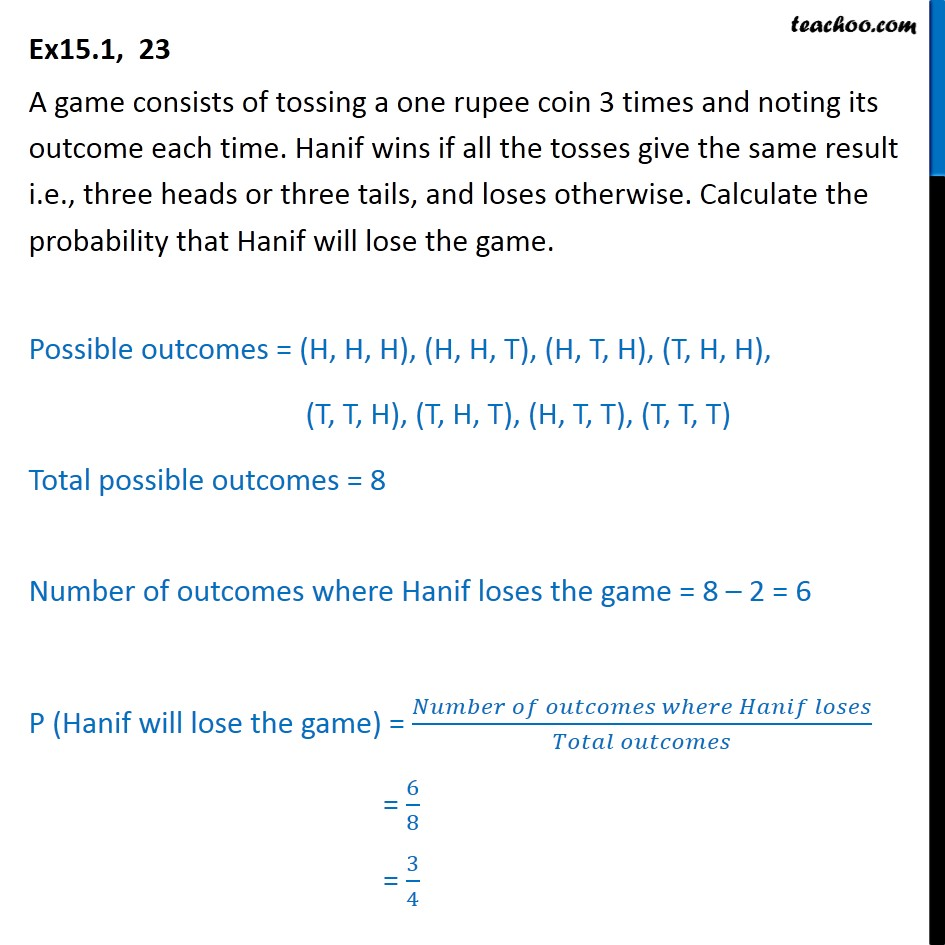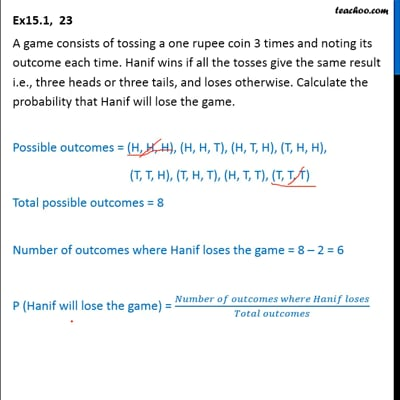Ex 15.1

Chapter 15 Class 10 Probability (Term 1)
Serial order wiseThis video is only available for Teachoo black users

### Transcript

Ex15.1, 23 A game consists of tossing a one rupee coin 3 times and noting its outcome each time. Hanif wins if all the tosses give the same result i.e., three heads or three tails, and loses otherwise. Calculate the probability that Hanif will lose the game. Possible outcomes = (H, H, H), (H, H, T), (H, T, H), (T, H, H), (T, T, H), (T, H, T), (H, T, T), (T, T, T) Total possible outcomes = 8 Number of outcomes where Hanif loses the game = 8 – 2 = 6 P (Hanif will lose the game) = (𝑁𝑢𝑚𝑏𝑒𝑟 𝑜𝑓 𝑜𝑢𝑡𝑐𝑜𝑚𝑒𝑠 𝑤ℎ𝑒𝑟𝑒 𝐻𝑎𝑛𝑖𝑓 𝑙𝑜𝑠𝑒𝑠)/(𝑇𝑜𝑡𝑎𝑙 𝑜𝑢𝑡𝑐𝑜𝑚𝑒𝑠) = 6/8 = 3/4